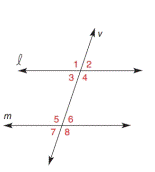Chapter 2.3, Problem 5EElementary Geometry For College St...

7th Edition
Alexander + 2 others
ISBN: 9781337614085

Solutions

Chapter
SectionElementary Geometry For College St...

7th Edition
Alexander + 2 others
ISBN: 9781337614085
Textbook Problem

In Exercises 1 to 6, l and m are cut by transversal v . On the basis of the information given, determine whether l must be parallel to m . m ∠ 3 = 113.5 ° and m ∠ 5 = 67.5 °To determine

To verify:

Whether 𝓁 must be parallel to m.

Explanation

Given:

𝓁 and m are cut by transversal v.

m3=113.5° and m5=67.5°.

Figure (1)

Concept:

(1) If two lines are cut by a transversal so that two alternate interior angles are not congruent, these lines are not parallel.

(2) The supplementary angles add up to 180°.

Approach:

The given statement is, 𝓁 and m are cut by transversal v.

m3=113.5° and m5=67.5°

6 and 5 are supplementary angles.

So, m6+m5=180°

Substitute 67.5° for m5 in the above equation

Still sussing out bartleby?

Check out a sample textbook solution.

See a sample solution

The Solution to Your Study Problems

Bartleby provides explanations to thousands of textbook problems written by our experts, many with advanced degrees!

Get Started

Evaluate the iterated integral: 0202cosrdrd

Calculus: Early Transcendental Functions

sec1(23)= a) 3 b) 3 c) 6 d) 6

Study Guide for Stewart's Single Variable Calculus: Early Transcendentals, 8th

j × (−k) = i −i j + k −j − k

Study Guide for Stewart's Multivariable Calculus, 8th

Explain how a researcher using simple random sampling can still obtain a biased sample.

Research Methods for the Behavioral Sciences (MindTap Course List)